# Speaker Recognition Using x-vectors

Speaker recognition answers the question "Who is speaking?". Speaker recognition is usually divided into two tasks: speaker identification and speaker verification. In speaker identification, a speaker is recognized by comparing their speech to a closed set of templates. In speaker verification, a speaker is recognized by comparing the likelihood that the speech belongs to a particular speaker against a predetermined threshold. Traditional machine learning methods perform well at these tasks in ideal conditions. For examples of speaker identification using traditional machine learning methods, see Speaker Identification Using Pitch and MFCC and Speaker Verification Using i-Vectors. Audio Toolbox™ provides `ivectorSystem` which encapsulates the ability to train an i-vector system, enroll speakers or other audio labels, evaluate the system for a decision threshold, and identify or verify speakers or other audio labels.

In adverse conditions, the deep learning approach of x-vectors has been shown to achieve state-of-the-art results for many scenarios and applications . The x-vector system is an evolution of i-vectors originally developed for the task of speaker verification.

In this example, you develop an x-vector system. First, you train a time-delay neural network (TDNN) to perform speaker identification. Then you train the traditional backends for an x-vector-based speaker verification system: an LDA projection matrix and a PLDA model. You then perform speaker verification using the TDNN and the backend dimensionality reduction and scoring. The x-vector system backend, or classifier, is the same as developed for i-vector systems. For details on the backend, see Speaker Verification Using i-Vectors and `ivectorSystem`.

In Speaker Diarization Using x-vectors, you use the x-vector system trained in this example to perform speaker diarization. Speaker diarization answers the question, "Who spoke when?".

Throughout this example, you will find live controls on tunable parameters. Changing the controls does not rerun the example. If you change a control, you must rerun the example.

### Data Set Management

This example uses a subset of the LibriSpeech Dataset . The LibriSpeech Dataset is a large corpus of read English speech sampled at 16 kHz. The data is derived from audiobooks read from the LibriVox project. Download the 100-hour subset of the LibriSpeech training data, the clean development set, and the clean test set.

```dataFolder = tempdir; datasetTrain = fullfile(dataFolder,"LibriSpeech","train-clean-100"); if ~datasetExists(datasetTrain) filename = "train-clean-100.tar.gz"; url = "http://www.openSLR.org/resources/12/" + filename; gunzip(url,dataFolder); unzippedFile = fullfile(dataFolder,filename); untar(unzippedFile{1}(1:end-3),dataFolder); end datasetDev = fullfile(dataFolder,"LibriSpeech","dev-clean"); if ~datasetExists(datasetDev) filename = "dev-clean.tar.gz"; url = "http://www.openSLR.org/resources/12/" + filename; gunzip(url,dataFolder); unzippedFile = fullfile(dataFolder,filename); untar(unzippedFile{1}(1:end-3),dataFolder); end datasetTest = fullfile(dataFolder,"LibriSpeech","test-clean"); if ~datasetExists(datasetTest) filename = "test-clean.tar.gz"; url = "http://www.openSLR.org/resources/12/" + filename; gunzip(url,dataFolder); unzippedFile = fullfile(dataFolder,filename); untar(unzippedFile{1}(1:end-3),dataFolder); end```

Create `audioDatastore` objects that point to the data. The speaker labels are encoded in the file names. Split the datastore into train, validation, and test sets. You will use these sets to train, validate, and test a TDNN.

```adsTrain = audioDatastore(datasetTrain,IncludeSubfolders=true); adsTrain.Labels = categorical(extractBetween(adsTrain.Files,fullfile(datasetTrain,filesep),filesep)); adsDev = audioDatastore(datasetDev,IncludeSubfolders=true); adsDev.Labels = categorical(extractBetween(adsDev.Files,fullfile(datasetDev,filesep),filesep)); adsEvaluate = audioDatastore(datasetTest,IncludeSubfolders=true); adsEvaluate.Labels = categorical(extractBetween(adsEvaluate.Files,fullfile(datasetTest,filesep),filesep));```

Separate the `audioDatastore` objects into five sets:

• `adsTrain` - Contains training set for the TDNN and backend classifier.

• `adsValidation` - Contains validation set to evaluate TDNN training progress.

• `adsTest` - Contains test set to evaluate the TDNN performance for speaker identification.

• `adsEnroll` - Contains enrollment set to evaluate the detection error tradeoff of the x-vector system for speaker verification.

• `adsDET` - Contains evaluation set used to determine the detection error tradeoff of the x-vector system for speaker verification.

```[adsTrain,adsValidation,adsTest] = splitEachLabel(adsTrain,0.8,0.1,0.1,"randomized"); [adsEnroll,adsLeftover] = splitEachLabel(adsEvaluate,3,"randomized"); adsDET = audioDatastore([adsLeftover.Files;adsDev.Files]); adsDET.Labels = [adsLeftover.Labels;adsDev.Labels];```

You can reduce the training and detection error trade-off datasets used in this example to speed up the runtime at the cost of performance. In general, reducing the data set is a good practice for development and debugging.

```speedupExample =false; if speedupExample adsTrain = splitEachLabel(adsTrain,5); adsValidation = splitEachLabel(adsValidation,2); adsDET = splitEachLabel(adsDET,5); end```

### Feature Extraction

Create an `audioFeatureExtractor` object to extract 30 MFCCs from 30 ms Hann windows with a 10 ms hop. The sample rate of the data set is 16 kHz.

```fs = 16e3; windowDuration =0.03; hopDuration =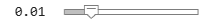0.01; windowSamples = round(windowDuration*fs); hopSamples = round(hopDuration*fs); overlapSamples = windowSamples - hopSamples; numCoeffs =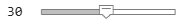30; afe = audioFeatureExtractor( ... SampleRate=fs, ... Window=hann(windowSamples,"periodic"), ... OverlapLength=overlapSamples, ... mfcc=true); setExtractorParameters(afe,"mfcc",NumCoeffs=numCoeffs)```

Create a transform datastore that applies preprocessing to the audio and outputs features. The supporting function, xVectorPreprocess, performs speech detection, extract features from regions of speech. When the parameter `Segment` is set to `false`, the detect regions of speech are concatenated together.

```adsTrainTransform = transform(adsTrain,@(x)xVectorPreprocess(x,afe,Segment=false,MinimumDuration=0.5)); features = preview(adsTrainTransform)```
```features = 1×1 cell array {30×363 single} ```

In a loop, extract all features from the training set. If you have Parallel Computing Toolbox™, then the computations are spread across multiple workers.

```numPar = numpartitions(adsTrain); features = cell(1,numPar); parfor ii = 1:numPar adsPart = partition(adsTrainTransform,numPar,ii); N = numel(adsPart.UnderlyingDatastores{1}.Files); f = cell(1,N); for jj = 1:N f{jj} = read(adsPart); end features{ii} = cat(2,f{:}); end```

Concatenate the features and then save the global mean and standard deviation in a struct. You will use these factors to normalize features.

```features = cat(2,features{:}); features = cat(2,features{:}); factors = struct("Mean",mean(features,2),"STD",std(features,0,2)); clear features f```

Create a new transform datastore for the training set, this time specifying the normalization factors and `Segment` as `true`. Now, features are normalized by the global mean and standard deviation, and then the file-level mean. The individual speech regions detected are not concatenated. The output is a table with the first variable containing feature matrices and the second variable containing the label.

```adsTrainTransform = transform(adsTrain,@(x,myInfo)xVectorPreprocess(x,afe,myInfo, ... Segment=true,Factors=factors,MinimumDuration=0.5), ... IncludeInfo=true); featuresTable = preview(adsTrainTransform)```
```featuresTable=3×2 table features labels _______________ ______ {30×142 single} 1034 {30×64 single} 1034 {30×157 single} 1034 ```

Apply the same transformation to the validation, test, enrollment, and DET sets.

```adsValidationTransform = transform(adsValidation,@(x,myInfo)xVectorPreprocess(x,afe,myInfo, ... Segment=true,Factors=factors,MinimumDuration=0.5), ... IncludeInfo=true); adsTestTransform = transform(adsTest,@(x,myInfo)xVectorPreprocess(x,afe,myInfo, ... Segment=true,Factors=factors,MinimumDuration=0.5), ... IncludeInfo=true); adsEnrollTransform = transform(adsEnroll,@(x,myInfo)xVectorPreprocess(x,afe,myInfo, ... Segment=true,Factors=factors,MinimumDuration=0.5), ... IncludeInfo=true); adsDETTransform = transform(adsDET,@(x,myInfo)xVectorPreprocess(x,afe,myInfo, ... Segment=true,Factors=factors,MinimumDuration=0.5), ... IncludeInfo=true);```

### x-vector Feature Extraction Model

In this example, you define the x-vector feature extractor model  as a layer graph and train it using a custom training loop. This paradigm enables you to preprocess the mini-batches and trim the sequences to a consistent length.

The table summarizes the architecture of the network described in  and implemented in this example. T is the total number of frames (feature vectors over time) in an audio signal. N is the number of classes (speakers) in the training set.Define the network. You can change the model size by increasing or decreasing the `numFilters` parameter.

```numFilters =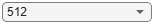512; dropProb = 0.2; numClasses = numel(unique(adsTrain.Labels)); layers = [ sequenceInputLayer(afe.FeatureVectorLength,MinLength=15,Name="input") convolution1dLayer(5,numFilters,DilationFactor=1,Name="conv_1") batchNormalizationLayer(Name="BN_1") dropoutLayer(dropProb,Name="drop_1") reluLayer(Name="act_1") convolution1dLayer(3,numFilters,DilationFactor=2,Name="conv_2") batchNormalizationLayer(Name="BN_2") dropoutLayer(dropProb,Name="drop_2") reluLayer(Name="act_2") convolution1dLayer(3,numFilters,DilationFactor=3,Name="conv_3") batchNormalizationLayer(Name="BN_3") dropoutLayer(dropProb,Name="drop_3") reluLayer(Name="act_3") convolution1dLayer(1,numFilters,DilationFactor=1,Name="conv_4") batchNormalizationLayer(Name="BN_4") dropoutLayer(dropProb,Name="drop_4") reluLayer(Name="act_4") convolution1dLayer(1,1500,DilationFactor=1,Name="conv_5") batchNormalizationLayer(Name="BN_5") dropoutLayer(dropProb,Name="drop_5") reluLayer(Name="act_5") statisticsPooling1dLayer(Name="statistics_pooling") fullyConnectedLayer(numFilters,Name="fc_1") batchNormalizationLayer(Name="BN_6") dropoutLayer(dropProb,Name="drop_6") reluLayer(Name="act_6") fullyConnectedLayer(numFilters,Name="fc_2") batchNormalizationLayer(Name="BN_7") dropoutLayer(dropProb,Name="drop_7") reluLayer(Name="act_7") fullyConnectedLayer(numClasses,Name="fc_3") softmaxLayer(Name="softmax") ]; dlnet = dlnetwork(layerGraph(layers));```

The model requires statistical pooling which is implemented as a custom layer and placed in your current folder when you open this example. Display the contents of the custom layer.

`type("statisticsPooling1dLayer.m")`
```classdef statisticsPooling1dLayer < nnet.layer.Layer & nnet.layer.Formattable % This class is only for use in this example. It may be changed or % removed in a future release. methods function this = statisticsPooling1dLayer(options) arguments options.Name = '' end this.Name = options.Name; end function X = predict(~, X) X = dlarray(stripdims([mean(X,3);std(X,0,3)]),"CB"); end function X = forward(~, X) X = X + 0.0001*rand(size(X),"single"); X = dlarray(stripdims([mean(X,3);std(X,0,3)]),"CB"); end end end ```

### Train Model

Use `minibatchqueue` (Deep Learning Toolbox) to create a mini-batch queue for the training data. Set the mini-batch size as appropriate for your hardware.

```miniBatchSize =256; mbq = minibatchqueue(adsTrainTransform, ... MiniBatchSize=miniBatchSize, ... MiniBatchFormat=["CTB",""], ... MiniBatchFcn=@preprocessMiniBatch, ... OutputEnvironment="auto");```

Set the number of training epochs, the initial learn rate, the learn rate drop period, the learn rate drop factor, and the validations per epoch.

```numEpochs =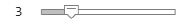5; learnRate =0.001; gradDecay = 0.5; sqGradDecay = 0.999; trailingAvg = []; trailingAvgSq = []; LearnRateDropPeriod =2; LearnRateDropFactor =0.1;```

To display training progress, initialize the supporting object `progressPlotter`. The supporting object, `progressPlotter`, is placed in your current folder when you open this example.

Run the training loop.

```classes = unique(adsTrain.Labels); pp = progressPlotter(string(classes)); iteration = 0; for epoch = 1:numEpochs % Shuffle mini-batch queue shuffle(mbq) while hasdata(mbq) % Update iteration counter iteration = iteration + 1; % Get mini-batch from mini-batch queue [dlX,Y] = next(mbq); % Evaluate the model gradients, state, and loss using dlfeval and the modelGradients function [gradients,dlnet.State,loss,predictions] = dlfeval(@modelGradients,dlnet,dlX,Y); % Update the network parameters using the Adam optimizer [dlnet,trailingAvg,trailingAvgSq] = adamupdate(dlnet,gradients, ... trailingAvg,trailingAvgSq,iteration,learnRate,gradDecay,sqGradDecay,eps("single")); % Update the training progress plot updateTrainingProgress(pp,Epoch=epoch,Iteration=iteration,LearnRate=learnRate,Predictions=predictions,Targets=Y,Loss=loss) end % Pass validation data through model [predictionValidation,labelsValidation] = predictBatch(dlnet,adsValidationTransform); predictionValidation = onehotdecode(predictionValidation,string(classes),1); % Update the training progress plot with validation results updateValidation(pp,Iteration=iteration,Predictions=predictionValidation,Targets=labelsValidation) % Update learn rate if rem(epoch,LearnRateDropPeriod)==0 learnRate = learnRate*LearnRateDropFactor; end end```Evaluate the TDNN speaker recognition accuracy using the held-out test set. The supporting function, `predictBatch`, parallelizes the prediction computation if you have Parallel Computing Toolbox™. Decode the predictions and then compute the prediction accuracy.

```[predictionTest,targetTest] = predictBatch(dlnet,adsTestTransform); predictionTest = onehotdecode(predictionTest,string(classes),1); accuracy = mean(targetTest(:)==predictionTest(:))```
```accuracy = 0.9460 ```

### Train x-vector System Backend

In the x-vector system for speaker verification, the TDNN you just trained is used to output an embedding layer. The output from the embedding layer ("`fc_1"` in this example) are the "x-vectors" in an x-vector system.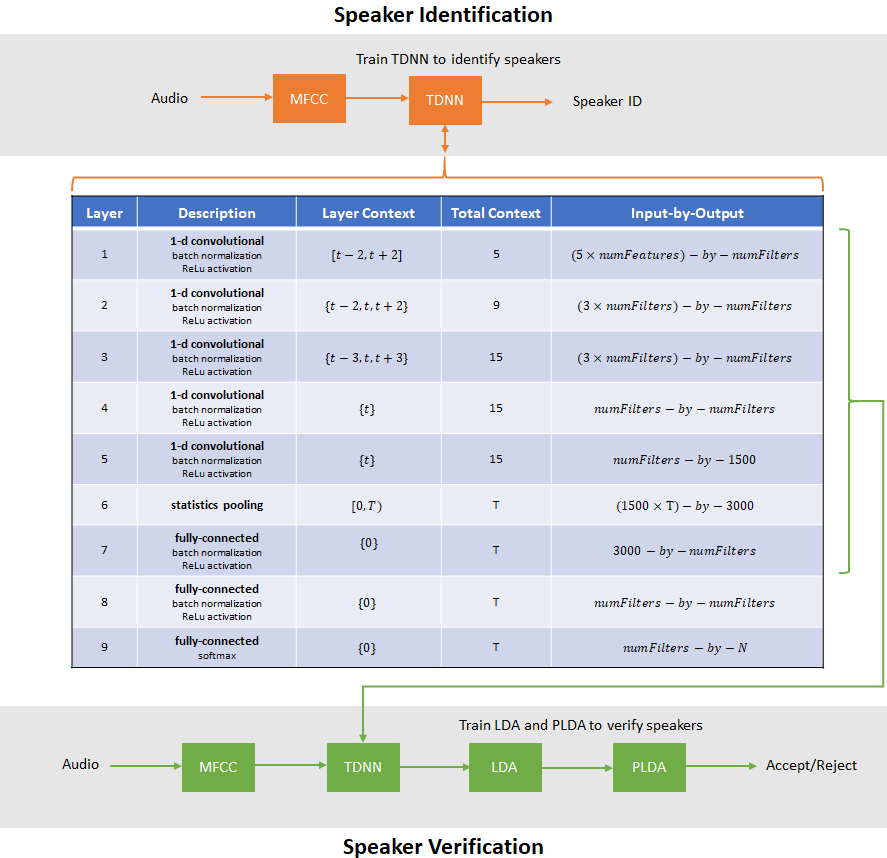The backend (or classifier) of an x-vector system is the same as the backend of an i-vector system. For details on the algorithms, see `ivectorSystem` and Speaker Verification Using i-Vectors.

Extract x-vectors from the train set.

`[xvecsTrain,labelsTrain] = predictBatch(dlnet,adsTrainTransform,Outputs="fc_1");`

Create a linear discriminant analysis (LDA) projection matrix to reduce the dimensionality of the x-vectors. LDA attempts to minimize the intra-class variance and maximize the variance between speakers.

```numEigenvectors =150; projMat = helperTrainProjectionMatrix(xvecsTrain,labelsTrain,numEigenvectors);```

Apply the LDA projection matrix to the x-vectors.

`xvecsTrainP = projMat*xvecsTrain;`

Train a G-PLDA model to perform scoring.

```numIterations =3; numDimensions =150; plda = helperTrainPLDA(xvecsTrainP,labelsTrain,numIterations,numDimensions);```

### Evaluate x-vector System

Speaker verification systems verify that a speaker is who they purport to be. Before a speaker can be verified, they must be enrolled in the system. Enrollment in the system means that the system has a template x-vector representation of the speaker.

#### Enroll Speakers

Extract x-vectors from the held-out data set, `adsEnroll`.

`[xvecsEnroll,labelsEnroll] = predictBatch(dlnet,adsEnrollTransform,Outputs="fc_1");`

Apply the LDA projection matrix to the x-vectors.

`xvecsEnrollP = projMat*xvecsEnroll;`

Create template x-vectors for each speaker by averaging the x-vectors of individual speakers across enrollment files.

```uniqueLabels = unique(labelsEnroll); enrollmentTable = cell2table(cell(0,2),VariableNames=["xvector","NumSamples"]); for ii = 1:numel(uniqueLabels) idx = uniqueLabels(ii)==labelsEnroll; wLocalMean = mean(xvecsEnrollP(:,idx),2); localTable = table({wLocalMean},(sum(idx)), ... VariableNames=["xvector","NumSamples"], ... RowNames=string(uniqueLabels(ii))); enrollmentTable = [enrollmentTable;localTable]; %#ok<AGROW> end```

Speaker verification systems require you to set a threshold that balances the probability of a false acceptance (FA) and the probability of a false rejection (FR), according to the requirements of your application. To determine the threshold that meets your FA/FR requirements, evaluate the detection error tradeoff of the system.

```[xvecsDET,labelsDET] = predictBatch(dlnet,adsDETTransform,Outputs="fc_1"); xvecsDETP = projMat*xvecsDET; detTable = helperDetectionErrorTradeoff(xvecsDETP,labelsDET,enrollmentTable,plda);```

Plot the results of the detection error tradeoff evaluation for both PLDA scoring and cosine similarity scoring (CSS).

```figure plot(detTable.PLDA.Threshold,detTable.PLDA.FAR, ... detTable.PLDA.Threshold,detTable.PLDA.FRR) eer = helperEqualErrorRate(detTable.PLDA); title(["Speaker Verification","Detection Error Tradeoff","PLDA Scoring","Equal Error Rate = " + eer]); xlabel("Threshold") ylabel("Error Rate") legend(["FAR","FRR"])``````figure plot(detTable.CSS.Threshold,detTable.CSS.FAR, ... detTable.CSS.Threshold,detTable.CSS.FRR) eer = helperEqualErrorRate(detTable.CSS); title(["Speaker Verification","Detection Error Tradeoff","Cosine Similarity Scoring","Equal Error Rate = " + eer]); xlabel("Threshold") ylabel("Error Rate") legend(["FAR","FRR"])```### References

 Snyder, David, et al. "x-vectors: Robust DNN Embeddings for Speaker Recognition." 2018 IEEE International Conference on Acoustics, Speech and Signal Processing (ICASSP), IEEE, 2018, pp. 5329–33. DOI.org (Crossref), doi:10.1109/ICASSP.2018.8461375.

 V. Panayotov, G. Chen, D. Povey and S. Khudanpur, "Librispeech: An ASR corpus based on public domain audio books," 2015 IEEE International Conference on Acoustics, Speech and Signal Processing (ICASSP), Brisbane, QLD, 2015, pp. 5206-5210, doi: 10.1109/ICASSP.2015.7178964

### Supporting Functions

#### Feature Extraction and Normalization

```function [output,myInfo] = xVectorPreprocess(audioData,afe,myInfo,nvargs) % This function is only for use in this example. It may be changed or % removed in a future release. arguments audioData afe myInfo = [] nvargs.Factors = [] nvargs.Segment = true; nvargs.MinimumDuration = 1; nvargs.UseGPU = false; end % Place on GPU if requested if nvargs.UseGPU audioData = gpuArray(audioData); end % Scale audioData = audioData/max(abs(audioData(:))); % Protect against NaNs audioData(isnan(audioData)) = 0; % Determine regions of speech mergeDur = 0.2; % seconds idx = detectSpeech(audioData,afe.SampleRate,MergeDistance=afe.SampleRate*mergeDur); % If a region is less than MinimumDuration seconds, drop it. if nvargs.Segment idxToRemove = (idx(:,2)-idx(:,1))<afe.SampleRate*nvargs.MinimumDuration; idx(idxToRemove,:) = []; end % Extract features numSegments = size(idx,1); features = cell(numSegments,1); for ii = 1:numSegments temp = (single(extract(afe,audioData(idx(ii,1):idx(ii,2)))))'; if isempty(temp) temp = zeros(30,15,"single"); end features{ii} = temp; end % Standardize features if ~isempty(nvargs.Factors) features = cellfun(@(x)(x-nvargs.Factors.Mean)./nvargs.Factors.STD,features,UniformOutput=false); end % Cepstral mean subtraction (for channel noise) if ~isempty(nvargs.Factors) fileMean = mean(cat(2,features{:}),"all"); features = cellfun(@(x)x - fileMean,features,UniformOutput=false); end if ~nvargs.Segment features = {cat(2,features{:})}; end if isempty(myInfo) output = features; else labels = repelem(myInfo.Label,numel(features),1); output = table(features,labels); end end```

#### Calculate Model Gradients and Updated State

```function [gradients,state,loss,YPred] = modelGradients(dlnet,X,Y) % This function is only for use in this example. It may be changed or % removed in a future release. [YPred,state] = forward(dlnet,X); loss = crossentropy(YPred,Y); gradients = dlgradient(loss,dlnet.Learnables); loss = double(gather(extractdata(loss))); end```

#### Preprocess Mini-Batch

```function [sequences,labels] = preprocessMiniBatch(sequences,labels) % This function is only for use in this example. It may be changed or % removed in a future release. trimDimension = 2; lengths = cellfun(@(x)size(x,trimDimension),sequences); minLength = min(lengths); sequences = cellfun(@(x)randomTruncate(x,trimDimension,minLength),sequences,UniformOutput=false); sequences = cat(3,sequences{:}); labels = cat(2,labels{:}); labels = onehotencode(labels,1); labels(isnan(labels)) = 0; end```

#### Randomly Truncate Audio Signals to Specified Length

```function y = randomTruncate(x,dim,minLength) % This function is only for use in this example. It may be changed or % removed in a future release. N = size(x,dim); if N > minLength start = randperm(N-minLength,1); if dim==1 y = x(start:start+minLength-1,:); elseif dim ==2 y = x(:,start:start+minLength-1); end else y = x; end end```

#### Predict Batch

```function [xvecs,labels] = predictBatch(dlnet,ds,nvargs) arguments dlnet ds nvargs.Outputs = []; end if ~isempty(ver("parallel")) pool = gcp; numPartition = numpartitions(ds,pool); else numPartition = 1; end xvecs = []; labels = []; outputs = nvargs.Outputs; parfor partitionIndex = 1:numPartition dsPart = partition(ds,numPartition,partitionIndex); partitionFeatures = []; partitionLabels = []; while hasdata(dsPart) atable = read(dsPart); F = atable.features; L = atable.labels; for kk = 1:numel(L) if isempty(outputs) f = gather(extractdata(predict(dlnet,(dlarray(F{kk},"CTB"))))); else f = gather(extractdata(predict(dlnet,(dlarray(F{kk},"CTB")),Outputs=outputs))); end l = L(kk); partitionFeatures = [partitionFeatures,f]; partitionLabels = [partitionLabels,l]; end end xvecs = [xvecs,partitionFeatures]; labels = [labels,partitionLabels]; end end```